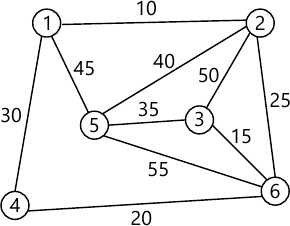MORE IN Data Structure & Algorithm Analysis
MU Information Technology (Semester 3)
Data Structure & Algorithm Analysis
December 2014
Total marks: --
Total time: --
INSTRUCTIONS
(1) Assume appropriate data and state your reasons
(2) Marks are given to the right of every question
(3) Draw neat diagrams wherever necessary

1 (a) Explain big O notation.
3 M
1 (b) Consider the following recursive function that takes two arguments
int foo (int n, int r)
{
if (n>0)
return ((n%r)+foo(n/r,r));
else
return 0;
}
What is the return value of the function foo when it is called as foo (65, 2)?
3 M
1 (c) What is queue? Specify ADT for it.
3 M
1 (d) What is linked list? State the different types of linked list.
3 M
1 (e) Write down properties of Red-Black tree.
3 M
1 (f) Define a graph. Which are the methos to represent a graph?
3 M
1 (g) Define minimum spanning tree. State the techniques to compute minimum spanning tree.
2 M

2 (a) Explain Quick sort using at example.
Write algorithm for it and comment on its complexity.
10 M
2 (b) Define double ended queue. Specify ADT for it. Implement any 2 operations of it.
10 M

3 (a) Construct the binary tree for the inorder and postorder traversal sequence given below:-
Inorder "INFORMATION"
Postorder "INFORMAINOTR"
Write a function to traverse a tree in postOrder.
10 M
3 (b) Convert following infix expression into prefix and postfix format. (a*b - (c+d//e^f)-g)*h
Write an algorithm Conversion () to convert infix expression into postfix expression.
10 M

4 (a) Write a program for implement array based Queue? List its applications.
10 M
4 (b) Sort the following data in descending order using Heap Sort.
20, 14, 50, 3, 5, 7, 11, 8, 12, 15
Show all the steps.
Write an algorithm for heap sort.
10 M

5 (a) What is an AVL tree? Construct AVL tree for following data. [Mention the type of rotation for each case.]
1,2,3,4,8,7,6,5,11,10,12.
10 M
5 (b) Write functions to implement insert () and traverse () of singly inked list.
10 M

6 (a) Draw the minimum cost spanning tree using Kruskal's algorithm. Also find its cost with all intermediate steps.10 M
6 (b) Explain Binary search tree with an example.
5 M
6 (c) Write an algorithm for DFS traversal.
5 M

More question papers from Data Structure & Algorithm Analysis